# Team:GreatBay SCIE/Optimum Aptamer Concentration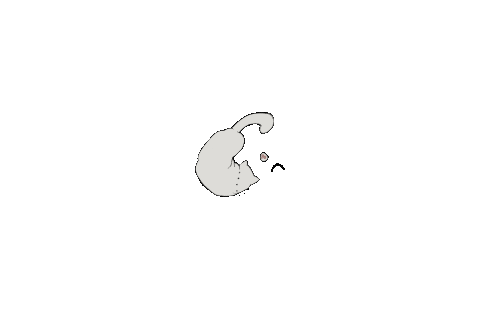<
Optimum liposome-aptamer ratio

# Introduction

The aim of this modeling is to utilize the least amount of aptamer to reach the highest binding affinity.

As a single liposome molecule can bind with numerous aptamers, we built up a mathematic model to estimate the best ratio between aptamer and liposome. Which we can use the least amount of aptamer to reach the highest binding affinity.

# Theory

At the beginning, we had to obtain some significant parameters.

We first use pymol(A mathematical modeling software) to measure the length between the epitopes on Her2 and cell surface which we estimate it as 5nm.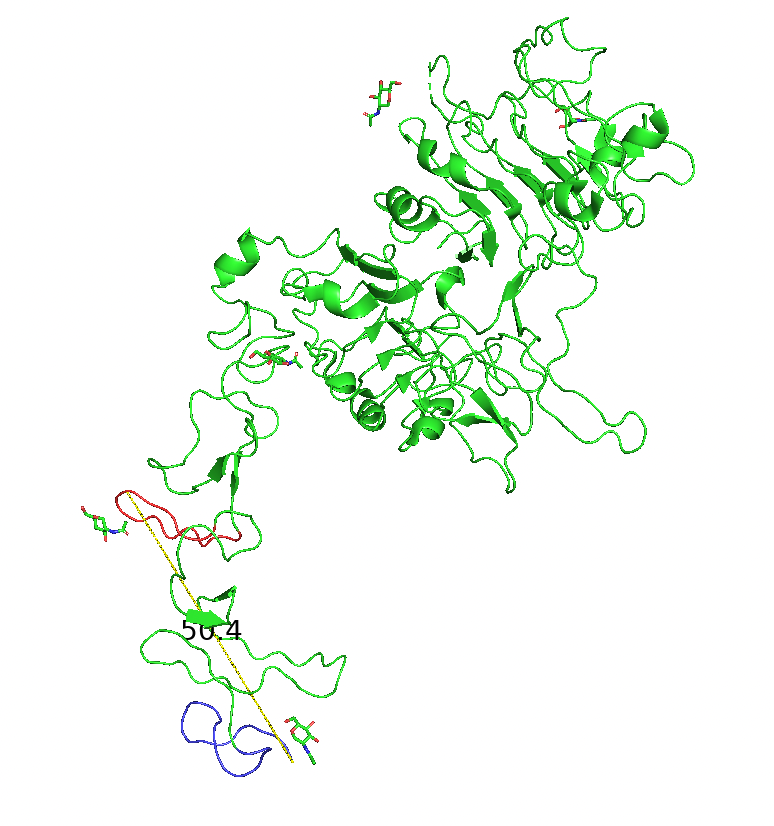Figure 1.1: The yellow line shows the distance between the sequence of epitope and the sequence on the cell membrane, which is 50.4, unit: Å

Thus two adjust aptamer on the liposome surface linear distance should be 10nm, as you can see in our schematic diagram below. The HER2 receptor can move around randomly around its conjugate sequence with the cell membrane to form a circle, the conjugate sequence which we labeled that sequence in our protein graph as blue. In this distance, no matter which part of the liposome collides with HER2, there is at least one aptamer that can attach with HER2.

By using the data from the liposome group, we know that the liposome we used has a radius of 158nm.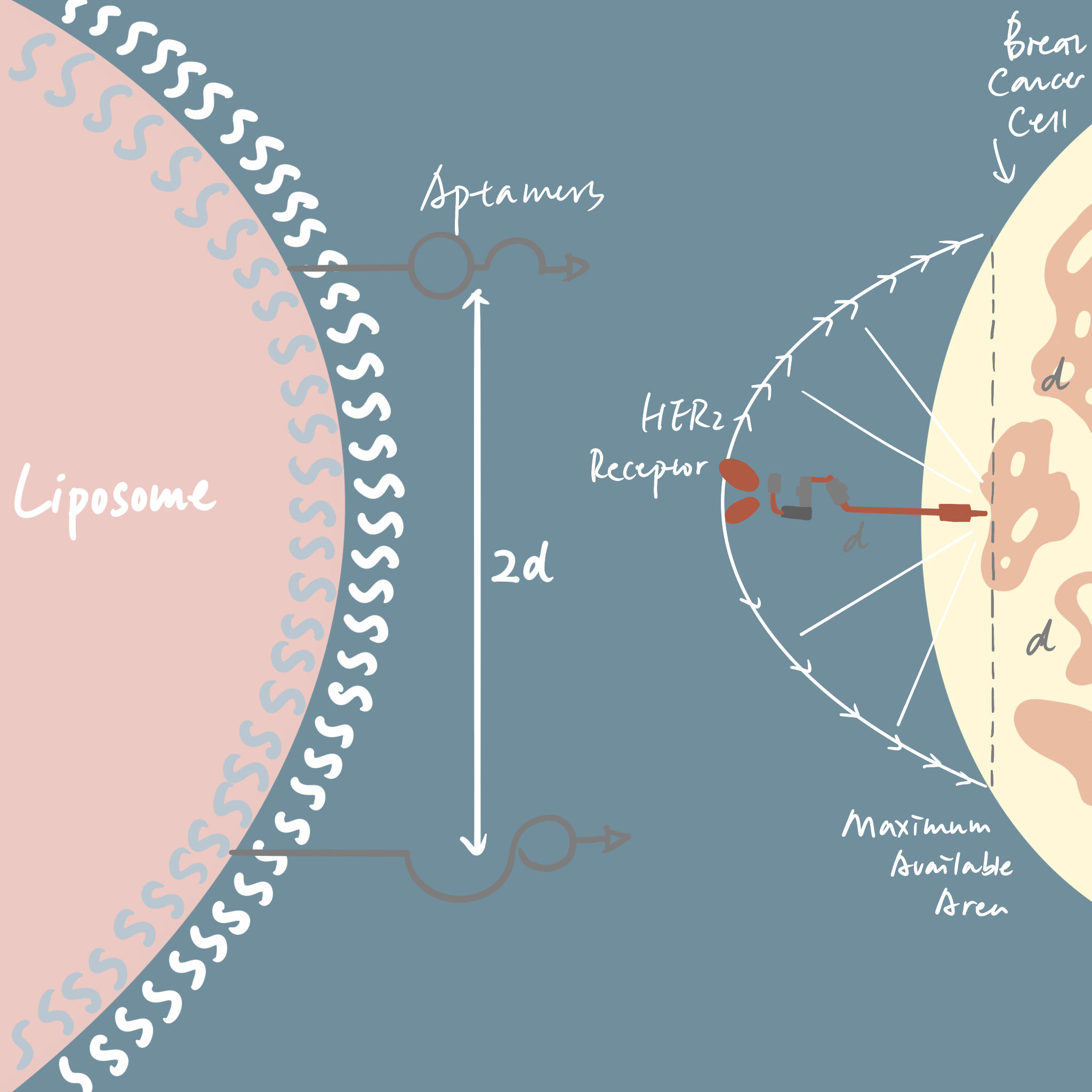Figure 1.2: The schematic diagram of why the distance between two adjust aptamer is 2d.

By using the law of cosine, we can obtain the intersection angle between two adjust aptamer

$$\frac{r^2+r^2-(2d)^2}{2r^2}=\cos\theta$$ $$\theta=\cos^{-1}(1-\frac{2d^2}{r^2})$$

Hence by using the definition of radian we can obtain the arc between two adjusted aptamer

$$S=\theta\times r$$

As the arc distance compared to the liposome radius is extremely small, hence we can assume the surface of the sphere can be cut into numerous hexagons. The side of the length of each hexagon is the length of the arc.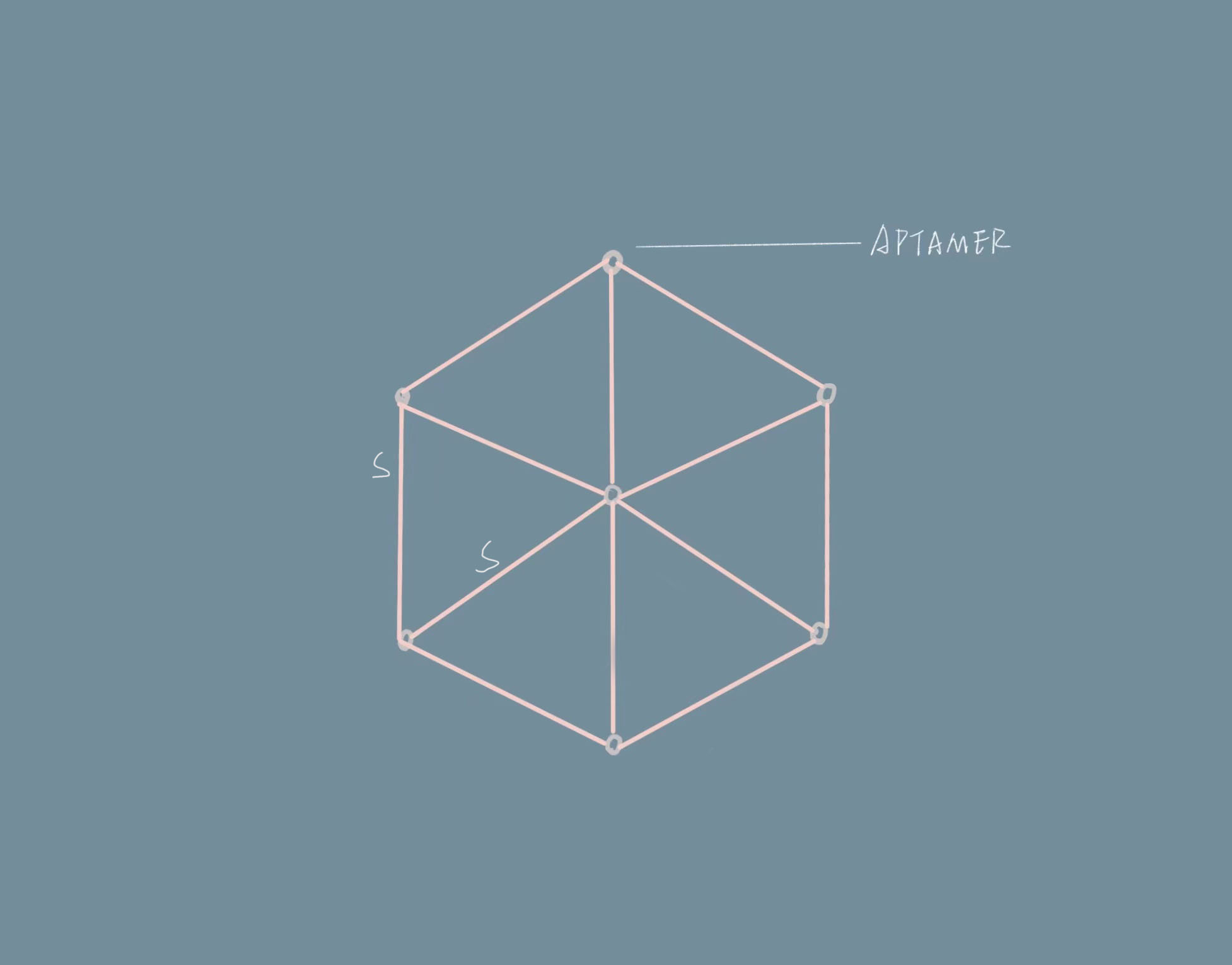Figure 1.3: The separation of aptamer can be considered as the hexagon

As the phospholipid has fluidity, thus the aptamer will separate on the surface of the liposome averagely. Therefore, we can assume that seven adjusted aptamer can form one equilateral hexagon separates on the sphere. One hexagon can be divided into six triangles. Then use the area of the sphere surface divided by of the equilateral triangle, we can obtain how many triangles are presented on its surface. Then times 3 as each triangle contain three vertexes, then divided by 6 because each aptamer acts as the vertex as well as the center. Therefore we can obtain the amount of the optimum aptamer number. Hence the ratio can be estimated.

$$(\frac{4\pi r^2}{\frac{\sqrt{3}}{4}S^2}\times\frac{1}{2}):1=n:1$$

(n is the number of the aptamer, S is the arc of two adjacent aptamers. r is the radius of the liposome. d is the length between an aptamer and cell membrane)

After we conjugated the aptamer and the drug we then designed an experiment based on ELISA to ensure our model is valid.

By using the data from the liposome group we can know that the radius of the liposome is r=158. Therefore we can calculate that the optimal ratio between this size of liposome and aptamer is about 1:3600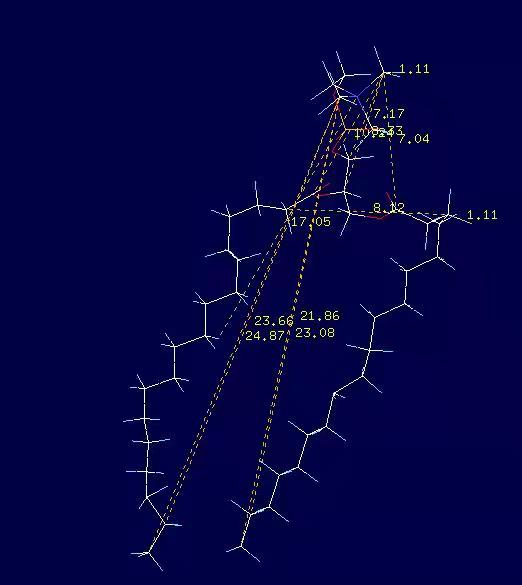Figure 1.4: The overview of a single phospholipid
a=8Å b=2Å

In order to make sure the aptamer can allow these number of binding, we generally calculate the maximum number of aptamer can bind with. Assume the surface of phospholipid is an ellipse, a represent the semi-major axis of the ellipse and b represent the semi-minor axis.

$$S_{area}=\pi\times \frac{a\times b}{4}$$

The ratio between phospholipid and PEG molecule is 10:1, so

$$N_{PEG}=\frac{4\pi r^2}{11S_{area}}$$

thus there is approximately 18060 individual PEG molecules on the surface of liposome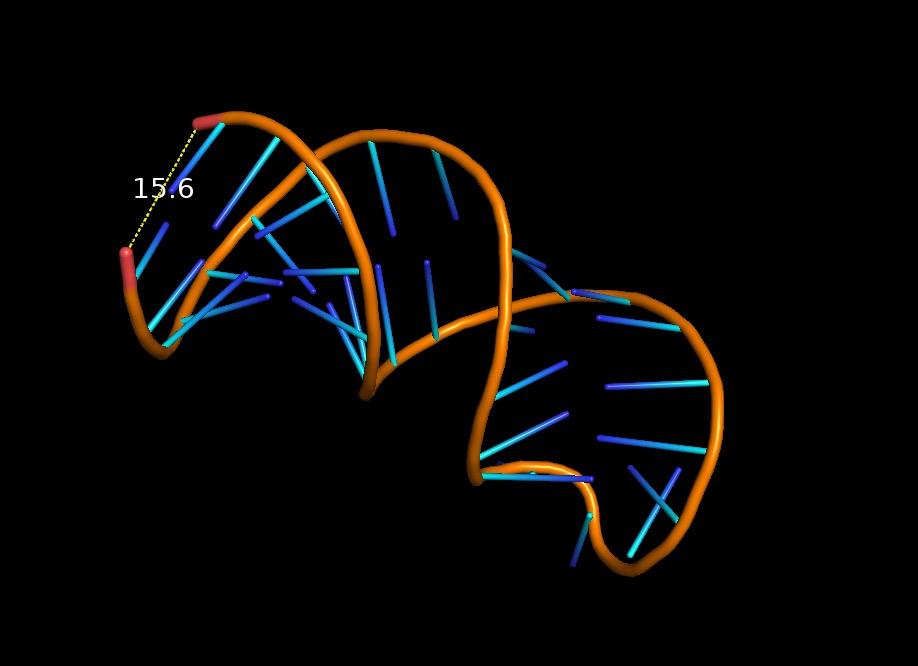Figure 1.5: The overview of the aptamer we used.

The width of the aptamer is 15.6Å, due to the steric effect, the surface area can be calculated, assuming each aptamer occupies a circle area. Using the sphere surface area divided by the occupied area by aptamer we can get the number of maximum aptamer of 10258

$$N_{APTAMER}=\frac{4\pi r^2}{4\pi 1.56^2}$$

As 10258<18060, so there are enough PEG molecules for an aptamer to bind with. Since the liposome can approximately tolerance the maximum number of 10258 individual aptamers. Hence we can conclude that the liposome can tolerate the aptamers of 3600, which is much smaller than the theoretical maximum.

# Experiment

The result of the experiment is shown on the graph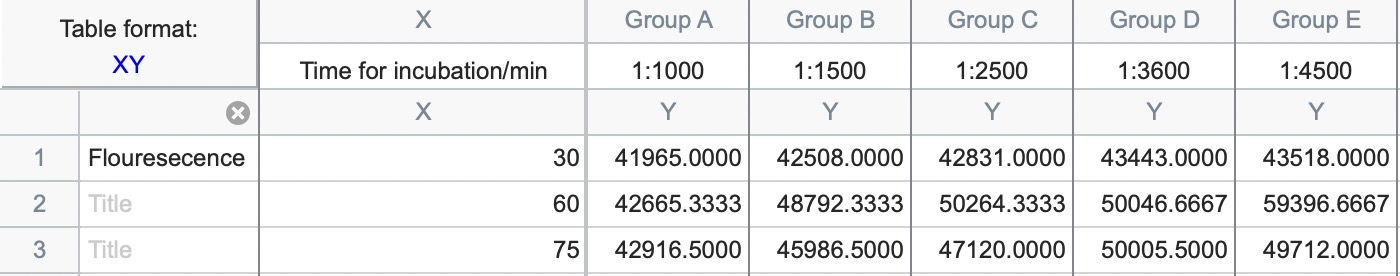Figure 1.6: The data of modified ELISA

We conduct the experiment by modifying the protocol of ELISA, in which we change the independent variable from concentration gradient to time gradient and get the result. You can click here to obtain more information in our protocol page.

Because we want the incubation time as the independent variable, therefore we added the same volume of liposome and different volumes of the aptamer. Since we assumed the aptamer can all bind with liposomes, hence the volume of aptamer won't affect the concentration. We only need to dilute the solution to reach the same volume hence the concentration can be controlled.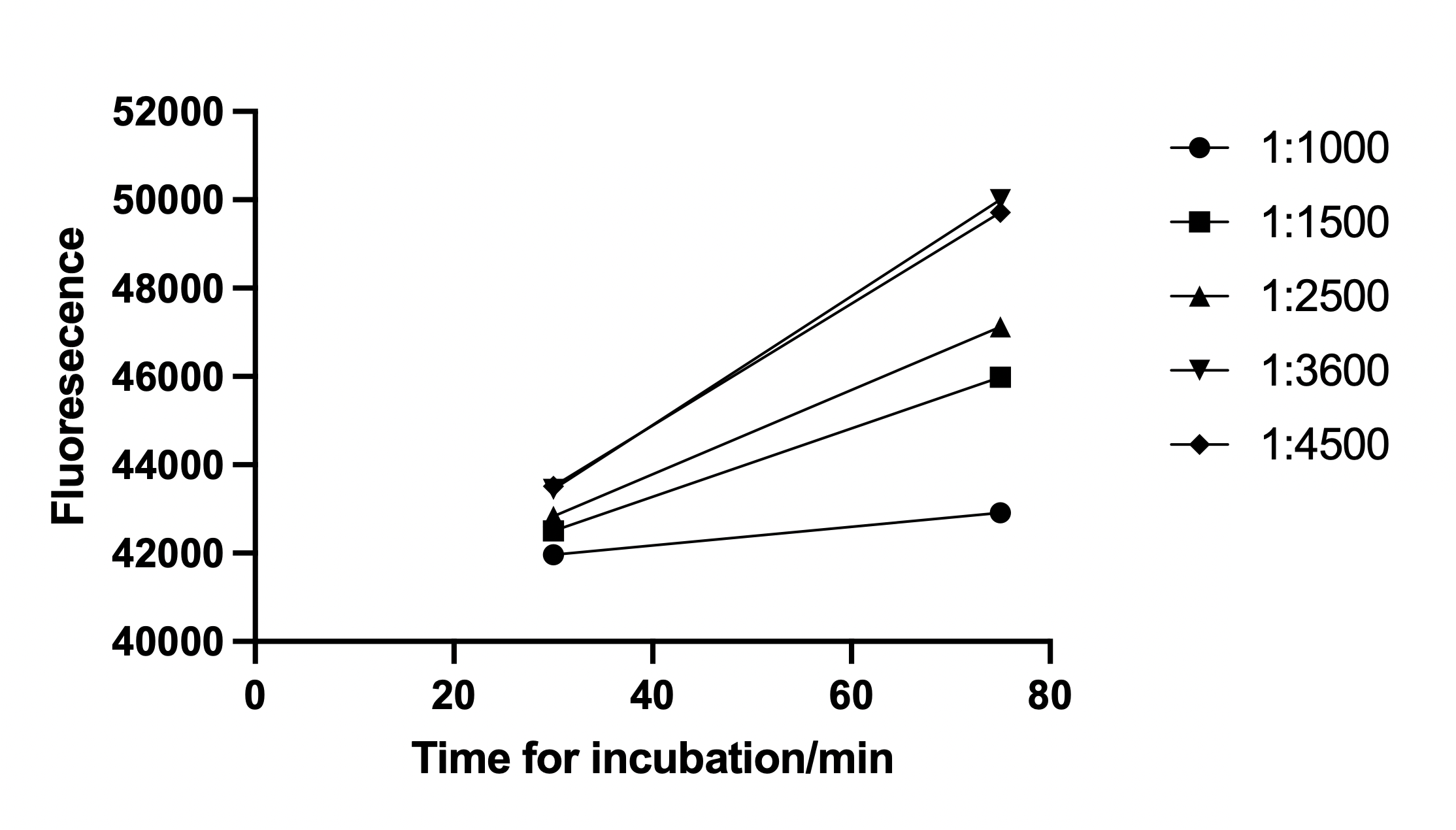Figure 1.6: The graph when incubation time is 30mins and 75 mins.

As you can see in our graph if only consider the incubation time at 30 minutes and 75 minutes(which is 15 minutes longer than the required incubation time), the fluorescence is extremely close between the ratio of 1:3600 and 1:4500, therefore the rate of binding is almost the same. This means by adding more aptamer on the liposome can't get a high binding affinity if the ratio is above 1:3600. Meanwhile, the ratio between 1: 1500 and 1:2500 shows a significantly lower fluorescence which is a lower binding rate. This can support our modeling is correct.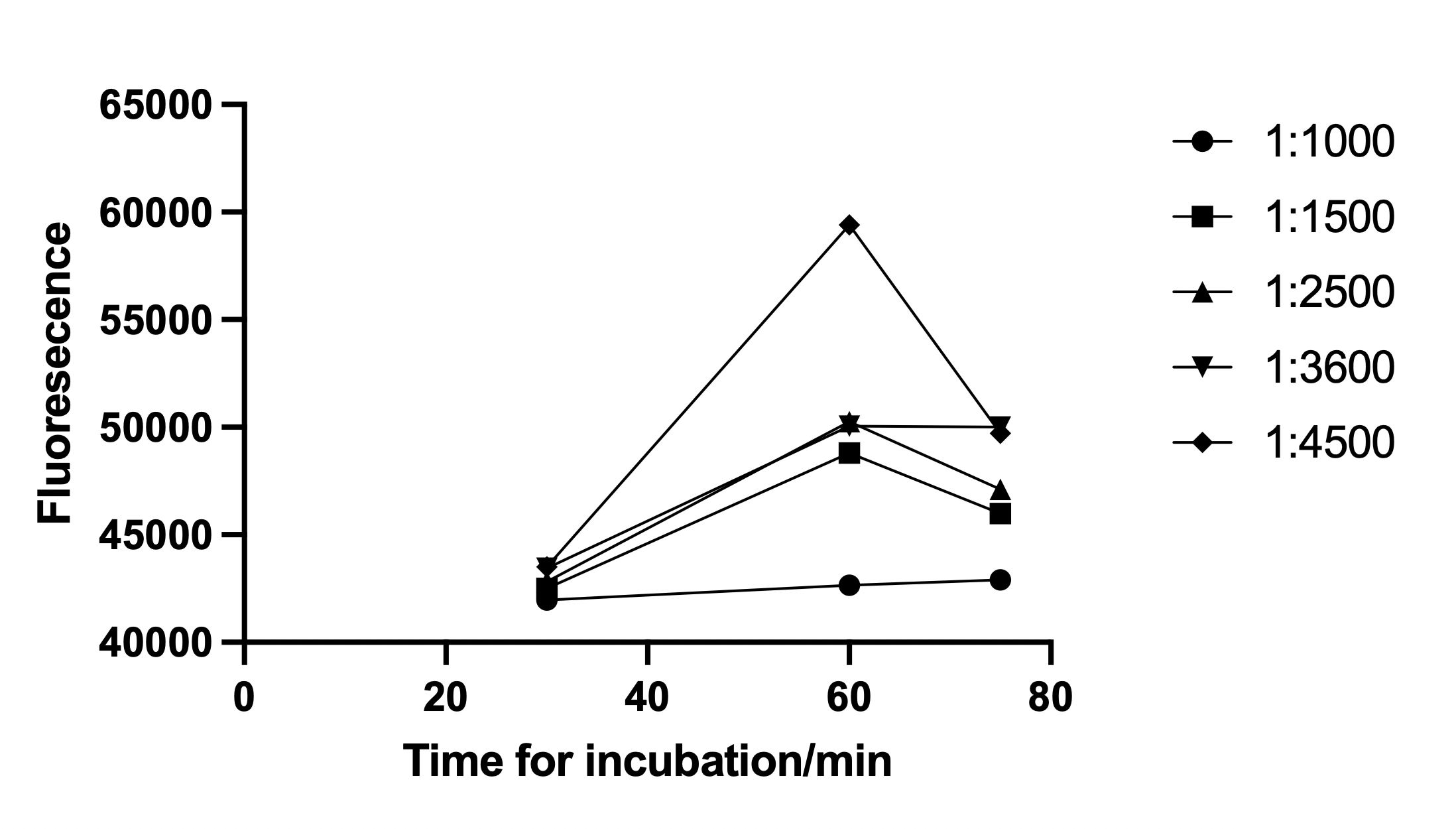Figure 1.7: The graph with incubation time of 30, 60, and 75 mins.

However, if we check the graph include the fluorescence at 60 minutes, there is a large increase in fluorescence, that can be caused by our manipulate error, as we placed the conjugated liposome-aptamer under room temperature for a long period of approximately 60 minutes and the liposome may decomposed before we add them for incubate, hence the concentration of aptamer is different in each group so the data in 60 minutes would be meaningless.

In order to check if the liposome from the 60 minutes group actually decomposed, we calculated its dissociate constant, Kd=0.4477 which is much higher than the other two group which 30mins group's Kd=0.05197 and 75mins group which Kd=0.2358. We can deduced from the data that the liposome has some decomposed hence our 60mins data might be the concentration gradient.